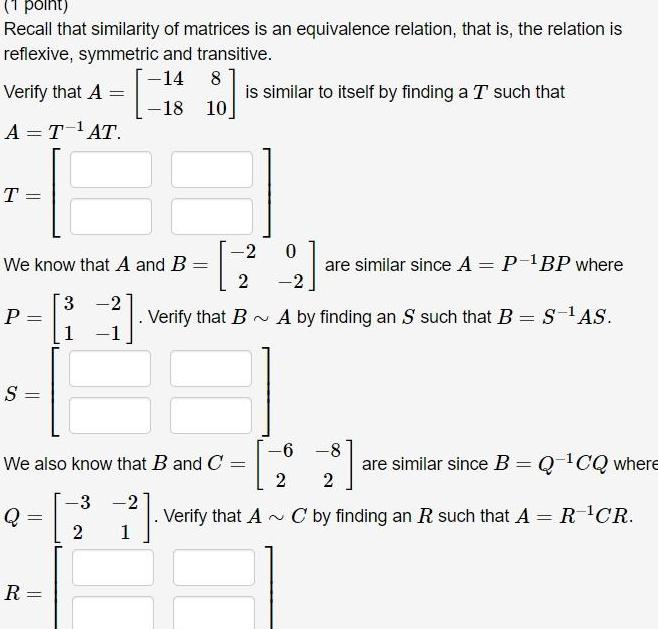Question:

# 1 point Recall that similarity of matrices is an equivalence

Last updated: 7/24/20231 point Recall that similarity of matrices is an equivalence relation that is the relation is reflexive symmetric and transitive 14 8 18 10 Verify that A A T AT T P S We know that A and B Q R 3 1 1 We also know that B and C 3 2 0 29 are similar since A P BP where Verify that BA by finding an S such that B S AS 2 1 is similar to itself by finding a T such that 6 8 2 2 Verify that A C by finding an R such that A R CR are similar since B Q CQ where